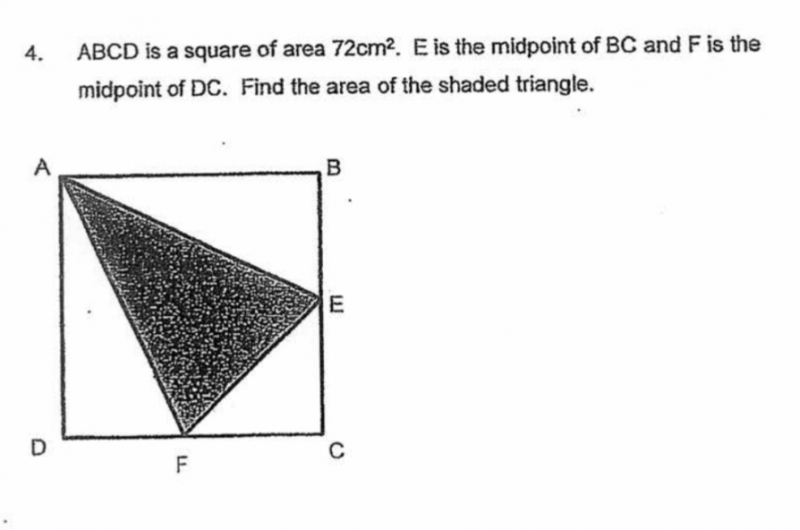# QuestionHi, we need help with this qn. Tq!

1 Answer

# Answer

DF = BE = 0.5 of 1 side of square

Area of triangle ADF = Area of triangle ABE

Total area of triangles ADF & ABE = 0.5 of area of square = 36 cm2

Area of triangle CEF = 1/8 of area of square = 72/ 8 = 9 cm2

Total shaded area = (72 – 36 – 9) cm2 = 27 cm2

2 Replies 1 Like ✔Accepted Answer

Hi , How do u know all the 1/8 and 0.5 of square.

Thanks in advance

0 Replies 0 Likes

Tq!

0 Replies 0 Likes